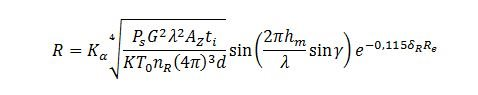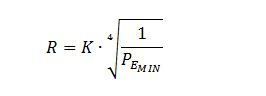# Increasing dynamic performance in radar systems

If you’re designing automotive radar or even commercial or military radar systems, we are all bound by physics.  I’d like to change that, but many of my old professors in college said “there are rules you can bend and then there are laws of nature that are immovable”.  Path loss in radio transmission is one of those things.  So, if you want your radar to see further, you need to improve the dynamic range of your system.

We’ll start with the basics to understand why dynamic range is an issue and where to look to improve it– the radar equation.  This defines how far the radar can see based on various system and atmospheric conditions.  The full (yikes!) equation is shown below (I’m having that math nightmare again!).But to see the effects on the system, we’ll strip it down to the basics… the classic radar equation.The first concern is obvious… the range is a function of the ratio of the transmitted power (PS)  including antenna gain and the target cross-section and the minimum power detectable by the receiver (PE[MIN]). This function is the fourth root, so assuming a fixed transmit power and target size while fixing the antenna gain and other constants the equation reduces to that shown below.Now we see the effect of improving the receiver’s dynamic range and sensitivity.  Improving the receiver’s noise power floor by a factor of 10 db (20 db in voltage noise) theoretically improves the range the radar sees by over 77%.

To fix this, consider the ENOB, or effective number of bits,of the system (not the data converter) that digitizes the returned signal.  There are several things that affect the noise floor such as  clock jitter (a form of noise that affects SINAD), quantization noise (the “bits” of resolution of the converter), and input referred noise of the amplifier chain.

Let’s start with the digitizer. For most modern radar systems, there is a down conversion stage before the data converters which are often utilized to digitize in the second Nyquist zone.  The ADS4449 is a 250 Msps 14 bit quad ADC with an SFDR of 87 db and a SINAD of over 70 db resulting in an ENOB of 11.5.  This is an excellent choice for many automotive or weather radar applications.

To get the signal to the data converter we’ll need a low noise mixing stage.  A good local oscillator (LO) synthesizer to consider is the TRF3765 which has extremely low RMS jitter on the order of 350 femtoseconds. To drive the ADC with the maximum dynamic range a high speed, low noise differential amplifier can be used which can utilize the common mode output of the ADC to place the input signal exactly where the ADC requires it. A good choice might be the LMH6552 which is designed to drive modern data converters.

And finally, but extremely important, is a master clock which is often supplied from an FPGA that grabs the output of the ADC. Depending on how far away the clock originates, or how much jitter is present, it’s a good idea to clean it before sending it to the ADC.  Remember, jitter is noise and directly affects the ENOB of the converter, so a clean clock is absolutely essential.  I’d suggest selecting a device from LMK048xx family such as the LMK04808.  These clock cleaners also have extremely low jitter and can restore the master clock as well as distribute the clean clock signals.

So, there you have it.  If you want your radar to see farther, you can use more transmit power, improve your antenna gain or simply use lower noise components in your receiver chain and improve the overall noise performance of the radar’s receiver.

If you’re interested in learning more about SINAD and ENOB, check out my colleague’s blog post called “SINAD, ENOB and the rest of the family”.

… till next time!

• OK… you asked for it… here are the parameter definitions for each equation

Equation 1:

-----------------------

Kα = Loss factor (combination of atmospheric loss, equipment loss, fluctuation loss during reflection, etc.)

PS = Transmitted Power (W)

G = Antenna Gain (V/V) - derived from antenna gain equation which includes transmitted wavelength.

λ = Transmitted Wavelength (m)

Az = Effective reflection surface (derived from radar cross section σ in m^2)

ti = Transmit Pulse length (S)

K = Boltzmann's constant (1.38 x 10-23 Watt*sec/°Kelvin)

T0 = absolute temperature (°Kelvin)

nR = Noise figure of the receiver

d = Clarity factor of the display terminal (note: residual parameter based on analog display technology – assume 1)

hm = Antenna height (m)

γ = Reflected beam angle

δR = Break-even factor

Re = Distance of the absorbing medium (m)

Equation 2 & 3:

------------------------

PEmin = Minimum Power Detectable by Receiver (W)

There are many "Radar Equations" for various applications... equation 1 in the blog is a "general" equation which includes atmospheric and other losses including antenna wave guides and couplings as well as target scattering. Equation 2 is stripped of many of these parameters to simplify the effects of receiver sensitivity - this is further reduced in equation 3.  Hope this helps...

The IEEE has some excellent on-line courses that describe these (and other) parameters in greater detail…

Source: ieeexplore.ieee.org/.../opac

Regards,

Rick Zarr

• please define symbols used in equations or provide reference for the equation(s).

thx

• Thank you. This is very nice post.